Q-Chem 4.3 User’s Manual

# 6.10 How to Compute Ionization Energies of Core Electrons and Excited States Involving Excitations of Core Electrons

In experiments using high-energy radiation (such as X-ray spectroscopy) core electrons can be ionized or excited to low-lying virtual orbitals. There are two ways to compute ionization energies of core electrons in Q-Chem. The first approach is a simple energy difference calculation in which core ionization is computed from energy differences computed for the neutral and core-ionized state. It is illustrated by this example:

Example 6.175  Q-Chem input for calculating chemical shift for 1-level of methane (CH). The first job is just an SCF calculation to obtain the orbitals and CCSD energy of the neutral. The second job solves the HF and CCSD equations for the core-ionized state.

$molecule 0,1 C 0.000000 0.000000 0.000000 H 0.631339 0.631339 0.631339 H -0.631339 -0.631339 0.631339 H -0.631339 0.631339 -0.631339 H 0.631339 -0.631339 -0.631339$end

$rem JOB_TYPE SP exchange HF CORRELATION CCSD basis 6-31G* MAX_CIS_CYCLES = 100$end

@@@
$molecule +1,2 C 0.000000 0.000000 0.000000 H 0.631339 0.631339 0.631339 H -0.631339 -0.631339 0.631339 H -0.631339 0.631339 -0.631339 H 0.631339 -0.631339 -0.631339$end

$rem JOB_TYPE SP UNRESTRICTED TRUE exchange HF basis 6-31G* MAX_CIS_CYCLES = 100 SCF_GUESS read Read MOs from previous job and use occupied as specified below CORRELATION CCSD MOM_START 1 Do not reorder orbitals in SCF procedure!$end

$occupied 1 2 3 4 5 2 3 4 5$end


In this job, we first compute the HF and CCSD energies of neutral CH: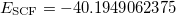and(HF orbital energy of the neutral gives Koopmans IE, which is 11.210 hartree = 305.03 eV). In the second job, we do the same for core-ionized CH. To obtain the desired SCF solution, MOM_START option and $occupied keyword are used. The resulting energies are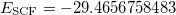(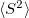= 0.7730) and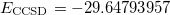. Thus,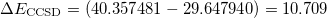hartree = 291.42 eV. This approach can be further extended to obtain multiple excited states involving core electrons by performing CIS, TDDFT, or EOM-EE calculations. The second approach is illustrated by the following input: Example 6.176 Q-Chem input for calculating chemical shift for 1-level of methane (CH) using EOM-IP. Here we solve SCF as usual, then reorder the MOs such that the core orbital becomes the “HOMO”, then solve the CCSD and EOM-IP equations with all valence orbitals frozen and the core orbital being active. $molecule
0,1
C      0.000000    0.000000    0.000000
H      0.631339    0.631339    0.631339
H     -0.631339   -0.631339    0.631339
H     -0.631339    0.631339   -0.631339
H      0.631339   -0.631339   -0.631339
$end$rem
JOB_TYPE SP
exchange HF
basis 6-31G*
MAX_CIS_CYCLES  = 100
CORRELATION CCSD
CCMAN2 = false
N_FROZEN_CORE 4 Freeze all valence orbitals
EOM_IP_STATES [1,0,0,0] Find one EOM_IP state
$end$reorder_mo
5 2 3 4 1
5 2 3 4 1
\$end


Here we use EOM-IP to compute core-ionized states. Since core states are very high in energy, we use “frozen core” trick to eliminate valence ionized states from the calculation. That is, we reorder MOs such that our core is the last occupied orbital and then freeze all the rest. The so computed EOM-IP energy is 245.57 eV. From the EOM-IP amplitude, we note that this state of a Koopmans character (dominated by single core ionization); thus, canonical HF MOs provide good representation of the correlated Dyson orbital. The same strategy can be used to compute core-excited states.

The accuracy of both calculations can be improved using triples corrections, e.g., CCSD(T) and EOM-IP-CCSD(dT). It is also recommend using a better basis that has more core functions.

## 6.10.1 Calculations of States Involving Core Electron Excitation/Ionization with DFT and TDDFT

TDDFT is not suited to describe the extended X-ray absorption fine structure (EXAFS) region, wherein the core electron is ejected and scattered by the neighboring atoms. Core-excitation energies computed with TDDFT with standard hybrid functionals are many electron volts too low compared with experiment. Exchange-correlation functionals specifically designed to treat core excitations are available in Q-Chem. These short-range corrected (SRC) functionals are a modification of the more familiar long-range corrected functionals (discussed in Section 4.3.4). However, in SRC-DFT the short-range component of the Coulomb operator utilizes predominantly Hartree-Fock exchange, while the mid to long-range component is primarily treated with standard DFT exchange. These functionals can be invoked by using the SRC_DFT rem. In addition, a number of parameters (OMEGA, OMEGA2, HF_LR, HF_SR) that control the shape of the short and long-range Hartree-Fock components need to be specified. Full details of these functionals and appropriate values for the parameters can be found in Refs. Besley:2009a,Besley:2010. An example of how to use these functionals is given below. For the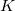-shell of heavy elements (2nd row of the periodic table) relativistic effects become increasing important and a further correction for these effects is required. Also calculations for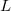-shell excitations are complicated by core-hole spin orbit coupling.